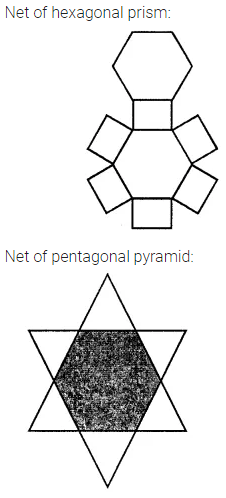# Selina Concise Mathematics Class 8 ICSE Solutions Chapter 19 Representing 3-D in 2-D

## Selina Concise Mathematics Class 8 ICSE Solutions Chapter 19 Representing 3-D in 2-D

Selina Publishers Concise Mathematics Class 8 ICSE Solutions Chapter 19 Representing 3-D in 2-D

### Representing 3-D in 2-D Exercise 19 – Selina Concise Mathematics Class 8 ICSE Solutions

Question 1.
If a polyhedron has 8 faces and 8 vertices, find the number of edges in it.
Solution: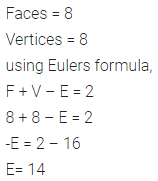Question 2.
If a polyhedron has 10 vertices and 7 faces, find the number of edges in it.
Solution: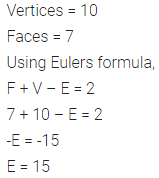Question 3.
State, the number of faces, number of vertices and number of edges of:
(i) a pentagonal pyramid
(ii) a hexagonal prism
Solution: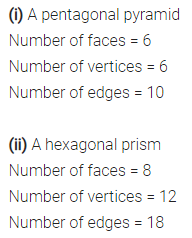Question 4.
Verily Euler’s formula for the following three dimensional figures:Solution: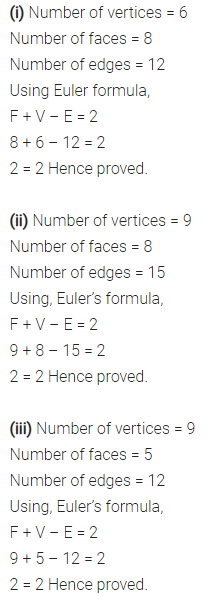Question 5.
Can a polyhedron have 8 faces, 26 edges and 16 vertices?
Solution:Question 6.
Can a polyhedron have:
(i) 3 triangles only ?
(ii) 4 triangles only ?
(iii) a square and four triangles ?
Solution:Question 7.
Using Euler’s formula, find the values of x, y, z.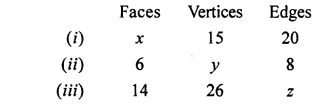Solution: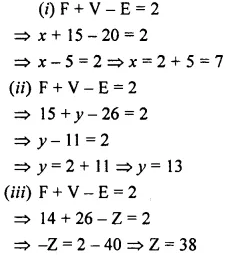Question 8.
What is the least number of planes that can enclose a solid? What is the name of the solid.
Solution:Question 9.
Is a square prism same as a cube?
Solution:Question 10.
A cubical box is 6 cm x 4 cm x 2 cm. Draw two different nets of it.
Solution: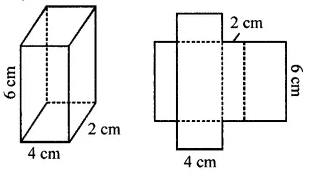Question 11.
Dice are cubes where the sum of the numbers on the opposite faces is 7. Find the missing numbers a, b and c.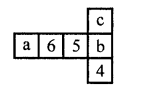Solution:Question 12.
Name the polyhedron that can be made by folding each of the following nets:Solution: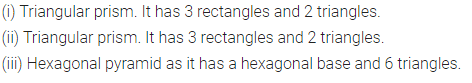Question 13.
Draw nets for the following polyhedrons: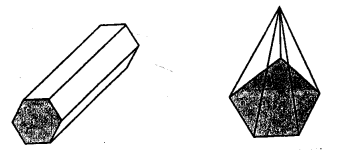Solution:
<